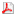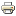Research Educational ActivitiesResearch activities at the Department encompass several main branches of pure and applied mathematics; namely algebra, topology, number theory, geometric analysis, mathematical analysis, statistics and mathematical modelling.

The Department provides teaching of professional mathematics (scholarly mathematics), mathematics aimed to finances and economy, and teaching of mathematics for future teachers in secondary schools. Our department also provides courses of mathematics for other branches at the Faculty of Science, such as physics, chemistry, biology, and geography. Furthermore, our members teach all main mathematical courses at the Faculty of Informatics and some mathematical courses at the Faculty of Economics and Administration. Our department has accreditation for the doctoral program of mathematics in

• algebra, number theory, and mathematical logic,
• geometry, topology, and global analysis,
• mathematical analysis,
• history of mathematics and mathematical education,
• probability, statistics, and mathematical modelling.

In cooperation with the university our department publishes the mathematical journal Archivum Mathematicum (http://emis.muni.cz/journals/AM/). Moreover, the Editorial Office of the journal Differential Geometry and its Applications (http://dga.math.muni.cz/) published by Elsevier is located at the department. Both journals are indexed in the international databases Mathematical Reviews, Zentralblatt für Mathematik, and in Scopus.

The following working groups are currently active at the department. The contact information for the employees is here, including the link to the publications, grant projects, and teaching activities.

#### ALGEBRA, TOPOLOGY AND NUMBER THEORY

Category theory (J. Rosický, L. Vokřínek, M. Lieberman). The research is mainly oriented to accessible categories and their applications to algebra, algebraic topology and model theory. Topics like Abstract elementary classes, Quillen model categories and weak factorization systems are included. We also deal with applications of abstract homotopy theory to computational topology.

Number theory (R. Kučera, M. Bulant). The research activity is focused on algebraic number theory, especially on abelian fields. The main aim is the investigation of algebraic structures having connection to the class group of these fields (like the group of circular units or Stickelberger ideal).

Ordered sets (J. Paseka, D. Kruml). The research deals with connections of algebra with logic, in particular quantum, tense and fuzzy. The basic tool are residuated posets but the emphasis is also on quantales in connection with C*-algebras and noncommutative geometry.

Semigroup theory (O. Klíma, M. Kunc, L. Polák). The research is focused on equational characterizations of pseudovarieties of finite semigroups and on lattices of pseudovarieties. We also deal with applications of semigroups to formal languages, for instance to the effective characterization of clases of regular languages.

#### GEOMETRIC ANALYSIS

Geometric complex analysis (Martin Kolář,  Ilya Kossovskiy). The research is focused on normal forms and invariants of  domains in complex space and their boundaries, in particular in the presence of singularities. The team is also interested in connections with the theory of dynamical systems.

Lie theory, geometric theory of partial differential equations and  geometric structures (Jan Slovák, Josef Šilhan, Matthew Randall, Ivan Minchev).  The aim is to use effectively the algebraic representation theory of Lie groups and algebras in geometric analysis for a large class of seemingly unrelated geometries (e.g. Projective, conformal, CR, quaternionic), properties of invariant differential operators for such geometries, connections with subriemannian geometry and direct applications in geometric control theory, computer vision and information geometry.

Modelling using partial differential equations, stochastics a spectral theory (Phan Thanh Nam) In many applications of mathematics, the basic models are based on such a large number of parameters, that in order to perform effecive computations, it is necessary to pass to nonlinear models with few parameters. This area of modern mathematical analysis lies on the border of deterministic and stochastic methods, which are used for description of large quantum systems.

Natural  operators and general geometric structures (Josef Janyška, Ivan Kolář, Jan Slovák). The main  object of interest of this group are general fibred spaces (jet bundles, Weil bundles, and natural bundles) invariant (i.e. geometric) operations on them, including those depending on special geometric structures, and applications of geometric methods in theoretical physics, in particular covariant classical and quantum theory on curved spacetime.

Geometric aspects of algebraic topology (Martin Čadek). The research concerns, on the one hand,  algorithmic approach to solution of classical problems of algebraic topology (description of homotopy classes of mappings, deciding about homotopy equivalence of two mappings, or two simplicial complexes), on the other hand topological conditions for existence of various geometric structures on low dimensional manifolds.

#### MATHEMATICAL ANALYSIS

Nonlinear differential and difference equations (Miroslav Bartušek, Zuzana Došlá, Petr Hasil, Josef Kalas, Michal Veselý). The research is focused to asymptotic theory of differential equations, including equations of delayed and neutral type. These equations have wide applications in natural sciences, medicine, and economy. We study  problems related to asymptotics, oscillation and stability of solutions, and boundary value problems on unbounded intervals, as well as their numerical discretizations. An important part of our research is the oscillation theory of Euler type half-linear differential and difference equations.

Limit periodic and almost periodic systems (Petr Hasil, Michal Veselý). The core of this research is the analysis of solutions of linear systems with non-periodic coefficients. Periodic differential and difference linear equations and systems (i.e., equations and systems with periodic inputs at the place of coefficients) belong to most frequently used continuous and discrete models of several (not only natural) processes. If the assumption of the pure periodicity is replaced by a more general case of limit periodicity or almost periodicity, specific functions and sequences (that cannot appear as solutions in a strictly periodic case) may be obtained as solutions of the considered equations and systems. This fact is consistent with observations in many situations, where periodic models do not allow a sufficiently accurate description of the studied phenomena. In the research, the emphasis is placed on studying those solutions that significantly differ from solutions of periodic systems, i.e., on the description of sets of non-almost periodic and non-asymptotically almost periodic solutions.

Hamiltonian and symplectic systems (Peter Šepitka, Roman Šimon Hilscher, Petr Zemánek). The main objects of our study are Hamiltonian differential systems and their discrete time counterpart symplectic systems – these systems are closely related with optimal processes in nature, science, and technology. We focus e.g. on the following particular topics: (i) structure of the systems, their perturbations and transformations, (ii) oscillation and asymptotic properties of solutions and the Sturm-Liouville theory, (iii) spectral properties of the systems and their associated operators and the Weyl-Titchmarsh theory, (iv) unified theory of continuous and discrete symplectic systems on time scales, and (v) applications in other fields of mathematics, such as in the calculus of variations and optimal control theory, LQR problems, etc.

Calculus of variations and optimal control (Roman Šimon Hilscher, Petr Zemánek). We investigate mathematical theory of optimality conditions of the first and second order in the calculus of variations and optimal control theory. Our reseach focuses for example on the following topics: necessary optimality conditions, sufficient optimality conditions, weak Pontryagin principle, isoperimetric problems or more generally problems with constraints, the Hamilton-Jacobi theory (dynamic programming), problems of linear-quadratic regulation (LQR problems), structure and solvability of Riccati equations. In our research we consider both continuous and discrete time optimization problems, as well as their unified theory on time scales.

#### MATEMATICAL  MODELLING AND STATISTICS

Matematical modelling (Zdeněk Pospíšil, Martin Kolář, Petr Lánský, Ondřej Pokora, Lenka Přibylová). Research activities are focused on  deterministic and  stochastic matematical  models of real processes, their analysis, numerical  simulations and computer  implementation (mathematical enviroments Matlab, Maple, R, Matcont, Xppaut). In particular, models  from the fields of  biomedicine, ecology, economy, epidemiology, neurophysiology  and engineering. Matematical models of  such processes  use mostly differential a diference equations, stochastic differential equations and metods of  numerical mathematics and statistics. Members of the team cooperate with, e.g.,  Brno University of Technology,   University of Defence,  FN Brno,  Biological center AV ČR, Institute of Physiology AV ČR, Department for the Study of Religions FF MU and Bicont Laboratory, s.r.o.

Statistics and data analysis (Ivana Horová, Marie Budíková, Marie Forbelská, Stanislav Katina, Jan Koláček, Andrea Kraus, David Kraus, Radim Navrátil,  Iveta Selingerová, Gejza Wimmer, Jiří Zelinka). The research of the team includes: parametric, nonparametric and robust statistical metods, kernel estimates of density,  regression function and risk function, survival analysis, time, space  and time-space  spline smoothing, shape and image analysis , statistical graphics, discrimination analysis, generalized linear models, statistical models for elliptical distributions, ROC curves and other techniques. The team cooperates with many institutions within the Czech republic and has extensive international cooperation,  e.g. with Institute of Clinical, Biological and Differential Psychology, Faculty of Psychology, University of Vienna;  School of Mathematics and Statistics, The University of Glasgow, Scotland,  Royal College of Surgeons in Ireland, Dublin, Ireland;  Ecole Polytechnique Fédérale de Lausanne; Centre Hospitalier Universitaire Vausois, Lausanne; Universität Bern;  Universitätsspital Bern;  Universität Zürich;  Universitätsspital Zürich.

#### OTHER ACTIVITIES:

In the area of mathematical education (Jaromír Šimša, Roman Plch)  we focus on problems of high school mathematics and preparation of its teachers during their undergraduate and postgraduate studies. A special attention is devoted to problem solving strategies designed for gifted high-school students and to teaching mathematics in an E-learning environment.

Last Updated on Tuesday, 30 May 2017 19:45